Study Guide

Remember back when we looked at linear inequalities? At one point, we said, "We promise we'll try to make your brain hurt more later." We always keep our promises, so grab an ice pack and strap in, because now we're going to look at quadratic inequalities.### Sample Problem

Solve x2 – 5x < -4.

When we solve an inequality, what we want are all the values of x that make the statement true. So our answers won't be single values, but large, sweeping regions of number space. You can then fence off those regions and raise cows on them.

We here at Shmoop love the equal sign. It's a good thing that the first step of solving an inequality is to pretend that the inequality is an equal sign. Set the equation "equal" to zero, and then solve to find the roots of the equation. They'll come in handy in a moment.

x2 – 5x < -4

x2 – 5x + 4 < 0

(x – 1)(x – 4) < 0

Okay, our roots are x = 1 and x = 4. So what? Take a look at the graph of this equation.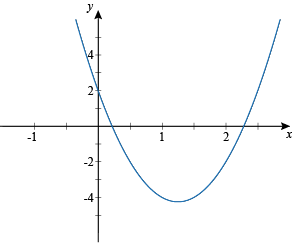A parabola is a smooth, continuous curve. The only places that it can possibly change sign (from above zero to below, or vice versa) are at the roots. We'll use this to help us find our solutions.

Hey, waitjustaminutehere! Couldn't we just graph the equation and solve it visually? We could, but there are two good reasons not to. First, it will often be just as or more difficult to graph the equation than it will be to solve it the other way (see: the next sample problem). Second, we can also use this technique to solve all kinds of polynomial inequalities, not just quadratic ones (see: the sample problem after that).

Anyway, back to solving. We'll now set up our roots on a number line, like so.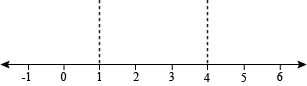We now have three regions fenced off. We need to pick a point from each region to check whether it's positive or negative within that region. Those regions that are negative will be our solutions (since our inequality ends with "< 0"). Afterwards we'll put our cows in the positive regions, to boost cow morale.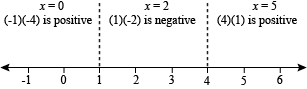All the values of x between 1 and 4 will cause the inequality to be true. So our solutions are 1 < x < 4. If you look back at the graph of the equation, you'll see that this is the region where it dips down below zero.

### Sample Problem

Solve -2x2 ≤ 6x + 1.

We again start off by getting all our stuff on one side of the inequality, leaving a big fat zero on the other side.

0 ≤ 2x2 + 6x + 1

2x2 + 6x + 1 ≥ 0

Last time we had a nice, factorable equation to work with. Not this time, bucko. Now we need to use the quadratic formula to find our roots.Our calculator tells us that these are x = -0.177 and x = -2.823. Now let's set up the number line and check the signs of each region.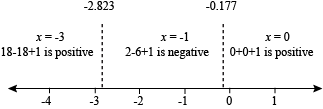We want the regions that are greater than zero this time, so the solutions are:

-∞ < x ≤ -2.823 and -0.177 ≤ x < ∞

So, is your brain starting to hurt? This next one is the last problem here, so stick with it a little longer.

### Sample Problem

Solve (x + 3)2(3x2 – 6) < 0.

This inequality definitely isn't quadratic, but the method for finding the solutions is the same. It's even factored already, making things way easier. The roots are x = -3,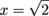, and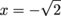. The number line looks like this:The expression is less than zero when -√2 < x < √2.

When working with polynomials larger than the quadratics there can be more than two roots, and we need to check the sign of every region. For every inequality, the sign won't necessarily follow a predictable pattern from one root to the next.

Is your brain throbbing with knowledge? That doesn't sound pleasant, but at least you're a bit smarter from the experience.